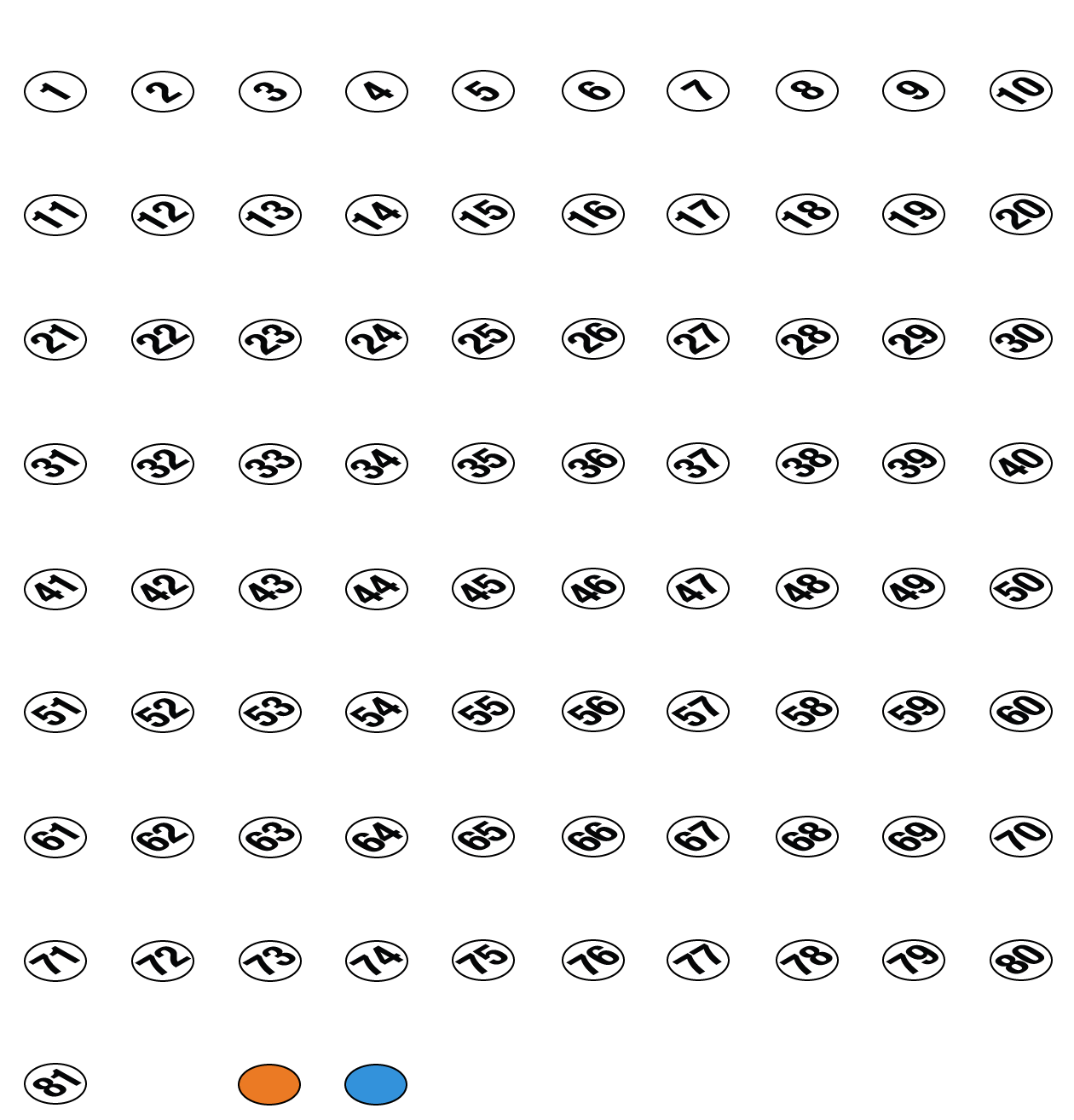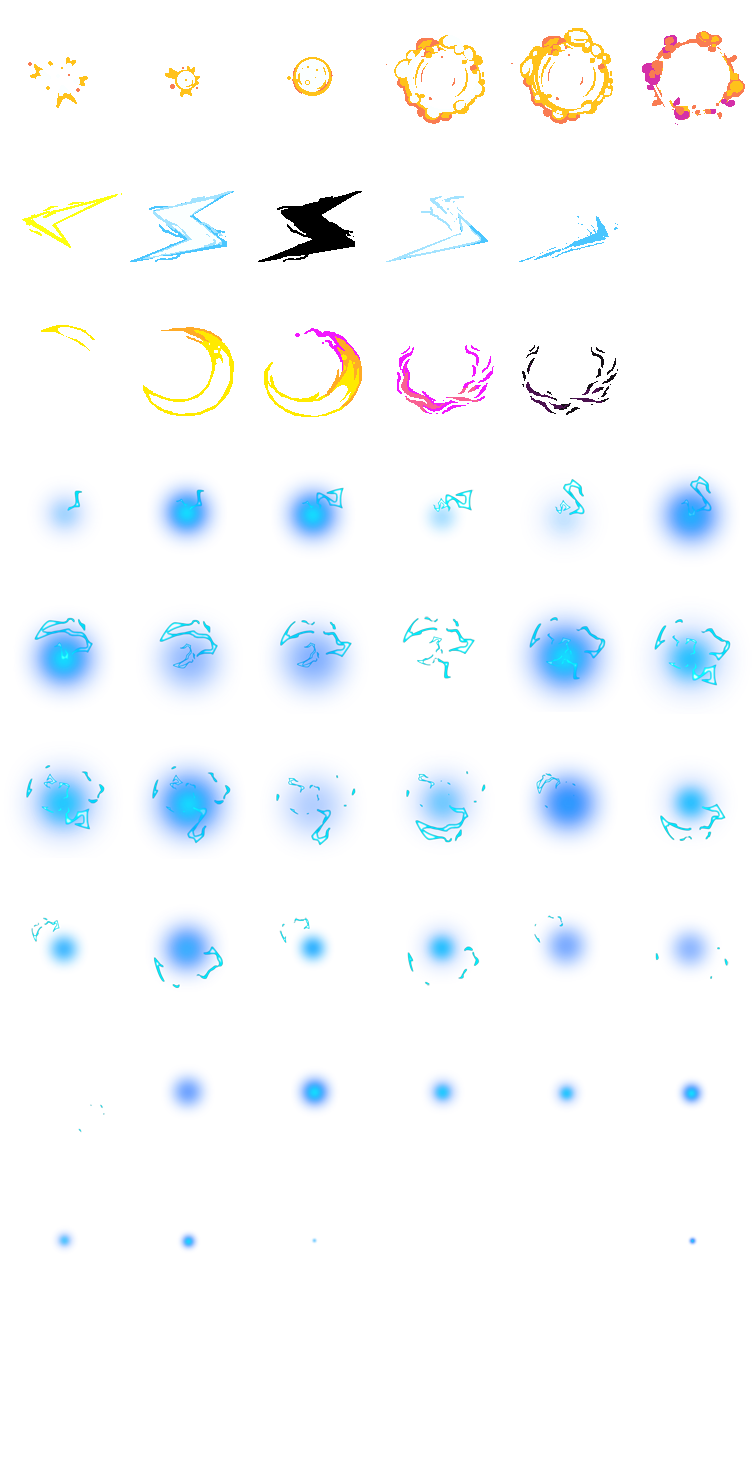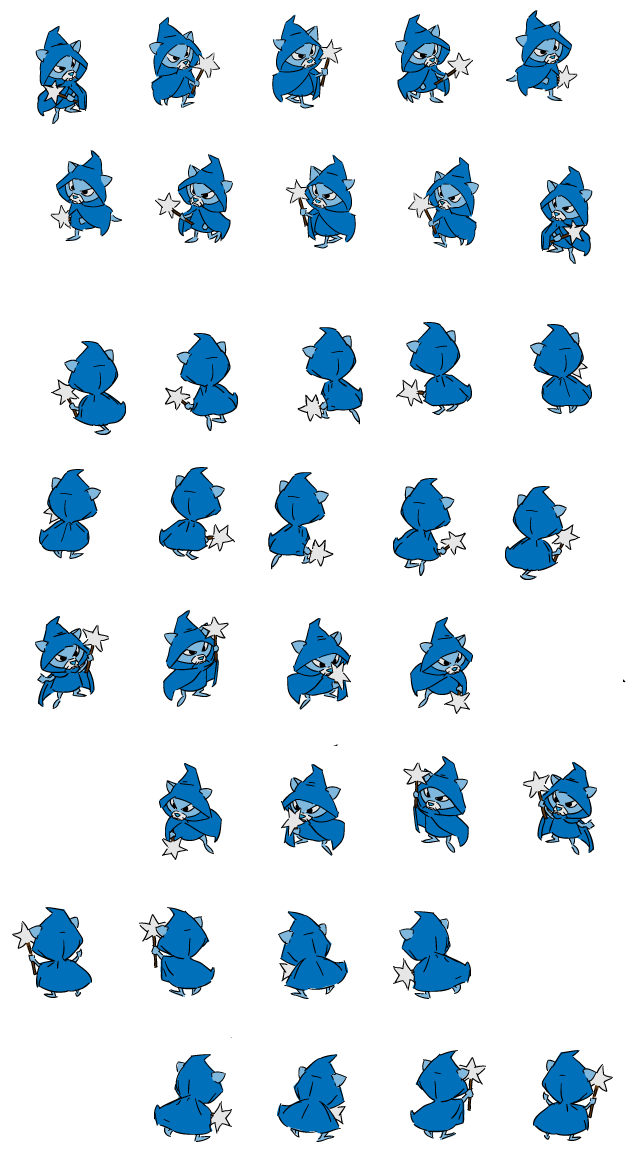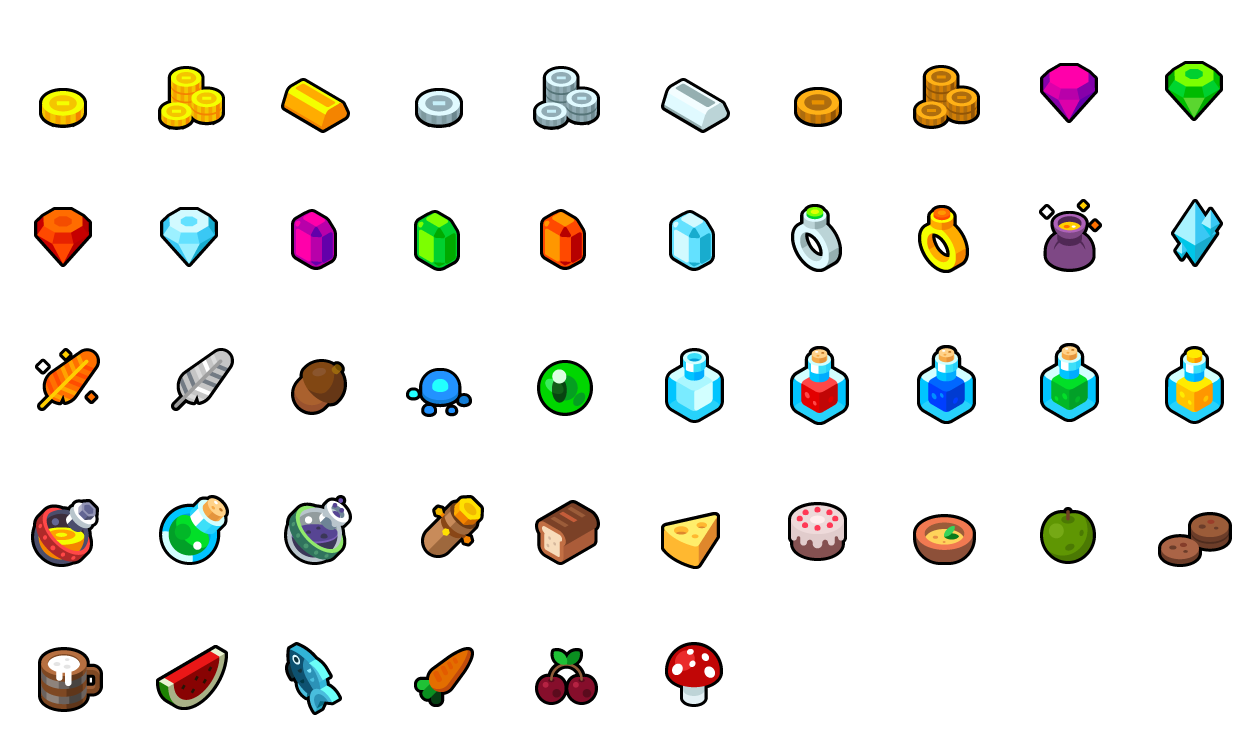i

## Magic Multiply# Magic Multiplication

Build math fluency with this fun online multiplication game for kids. Solve the multiplication equation by clicking on the correct number before the timer runs out. Kilala will cast a magic spell and reward you with treasure if you get it right!  Free to play, priceless for learning the times tables!

#### Common Core

Grade 3 >> CCSS.MATH.CONTENT.3.OA.C.7
Fluently multiply and divide within 100, using strategies such as the relationship between multiplication and division (e.g., knowing that 8 × 5 = 40, one knows 40 ÷ 5 = 8) or properties of operations. By the end of Grade 3, know from memory all products of two one-digit numbers.

i